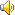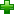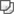# calculate ['kælkjuleit]# 英汉解释

vi. 计算；以为；作打算
vt. 计算；预测；认为；打算

# 词汇辨析

calculate，count，compute，reckon
这些动词均含有“计算”之意。
calculate   通常指要求细致精确和复杂的计算，以解决疑难问题，多用于自然科学、生产部门或要求专门计算的场合。
count   指逐一计算而得出总数。
compute   一般指已有数据的，较简单但数字长的数学运算。
reckon   通常指较简单的数学计算，也可指心算。

# 英英解释

v.
1. make a mathematical calculation or computation
2. judge to be probable
3. keep an account of
5. specifically design a product, event, or activity for a certain public
6. have faith or confidence in

# 网络释义

calculate
计算; 考虑 计划; 推测; 计算 推测;
calculate on
指望着; 预期，指望; 指望; 期待;
calculate off
停止; 转移; 取消;
calculate down
下计;
calculate up
上计;